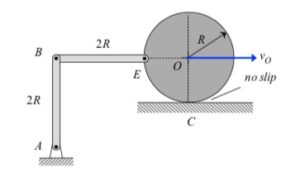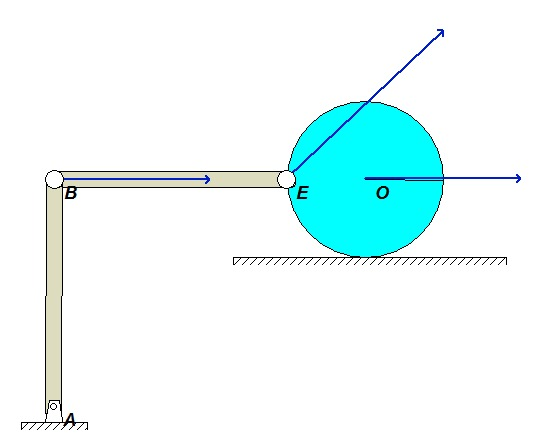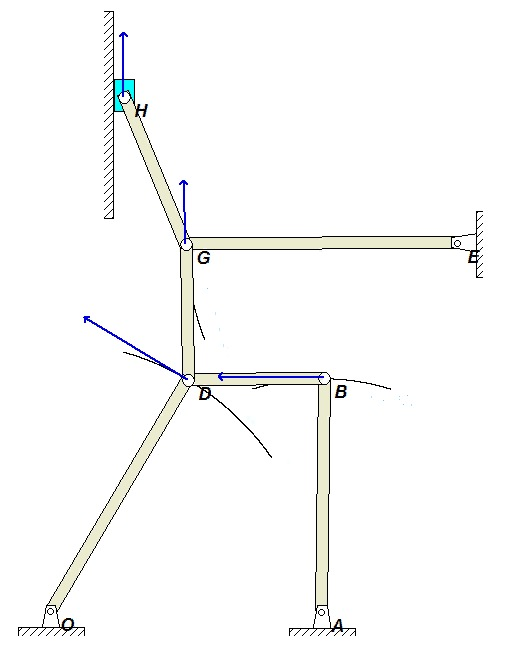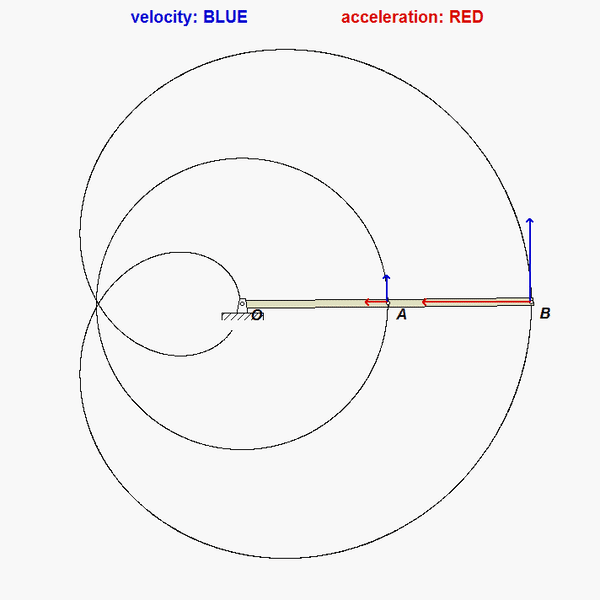# Homework H2.I - Fa23

 Problem statement Solution videoDISCUSSION
Suggested solution strategy:

• vA = vO + ωOA x rA/O
• vB = vH + ωBH x rB/H
• vA = vB + ωAB x rA/B
• Combine above, solving for the two unknown angular velocities.
• vE = vB + ωAB x rE/B# Homework H2.J - Fa23

 Problem statement Solution videoDISCUSSION
Suggested solution strategy:

• vB = vE + ωBE x rB/E
• vA = vO + ωAO x rA/O
• vA = vB + ωAB x rA/B
• Combine above, solving for the two unknown angular velocities.
• Repeat the above for accelerations.# Homework H2.G - Fa23

 Problem statement Solution videoDISCUSSION

• The no-slip point C is the IC for the disk. Therefore, the velocity of E, vE, is perpendicular to the line EC.
• AB rotates about the fixed point A. Therefore, the velocity of B, vB, is perpendicular to line AB.
• Construct perpendiculars to vE and vB.
• The intersection of these two perpendiculars is the IC for BE.In a freeze-frame of the simulation results below, note that vE is perpendicular to line EC, as expected.# Homework H2.H - Fa23

 Problem statement Solution videoDISCUSSION
Suggested solution strategy:

• Find IC for DB at the intersections of the velocities for D and B. Call this point K. In which direction is link DB rotating? And, in which direction is AB rotating?
• Find IC for DG at the intersections of the velocities for D and G. Call this point N. In which direction is link DG rotating? And, in which direction is EG rotating?
• Find IC for GH at the intersections of the velocities for G and H. In which direction is GH rotating?In a freeze-frame of the simulation results below, note the direction of motion for points D, B, G, E and H. Do these directions agree with those found in your analysis?# Homework H2.E - Fa23

 Problem statement Solution videoDISCUSSION
For velocity analysis, you will need a rigid body velocity equation for the triangular plate:

vA = vB + ωAB x rA/B

Take note that A and B both have constrained motion along their respective slots.# Homework H2.F - Fa23

 Problem statement Solution videoDISCUSSION
For velocity analysis, you will need a rigid body velocity equation for each link:
vB = vO + ωOB x rB/O
vA = vB + ωAB x rA/B

For acceleration analysis, you will need a rigid body acceleration equation for each link:
aB = aO + αOB x rB/OωOB2 rB/O
aA = aB + αAB x rA/B - ωAB2 rA/B

Take note that A has constrained motion along the inclined slot.# Homework H2.C - Fa23

 Problem statement Solution videoDISCUSSION
The tank is known to roll without slipping on the bed of the truck, with both the truck and the center of the tank C moving with constant speeds. Let D represent the contact point of the tank on the bed of the truck. As you write down the acceleration equation for point A referenced to the no-slip point D:
aA = aDα x rA/D - ω2rA/D
do not forget that the contact point D has a vertical component of acceleration.

# Homework H2.D - Fa23

 Problem statement Solution videoDISCUSSION
For velocity analysis, you will need a rigid body velocity equation for each link:
vA = vB + ωAB x rA/B
vA = vC + ω
disk x rA/C
and, for acceleration analysis you will need a rigid body acceleration equation for each link:
aA = aBαABx rA/B- ωAB2rA/B
aA = aC + α
disk x rA/C - ωdisk2rA/C
In addition for acceleration, you also need a rigid body acceleration equation relating points O and C on the disk.# Homework H2.A - Fa23

 Problem statement Solution videoDISCUSSION
Here you need to use the rigid body velocity and acceleration equations to relate the motions of points O and A:

vA = vO + ω x rA/O
aA = aO
α x rA/O - ω2rA/O

# Homework H2.B - Fa23

 Problem statement Solution videoDISCUSSION
For velocity analysis, you will need a rigid body velocity equation for each of the two links:
vA = vO + ωOA x rA/O
vB = vA + ω ABx rB/A
Combine these equations to get a single vector equation for the velocity of B.

Repeat the process for acceleration:
aA = aO
αOA x rA/O - ωOA2rA/O
aB = aA + αAB x rB/A - ωAB2rB/A